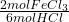Fe2O3 + 6HCl → 2FeCl3 + 6H20 How many atoms of FeCl3 will be produced if 9.3 x 1023 atoms of HCl are used for this reaction? 6.7 x 1023 atom

Question

Fe2O3 + 6HCl → 2FeCl3 + 6H20 How many atoms of FeCl3 will be produced if 9.3 x 1023 atoms of HCl are used for this reaction? 6.7 x 1023 atoms FeCl3 3.1 x 1023 atoms FeCl3 9.3 x 1023 atoms FeCl3 1.2 x 1023 atoms FeCl3​

in progress 0
5 months 2021-08-19T04:43:41+00:00 1 Answers 0 views 0

3.1×10²³

Explanation:

I’m going to answer the question assuming the number of molecules of FeCl₃ is asked, rather than the number of atoms.

• Fe₂O₃ + 6HCl → 2FeCl₃ + 6H₂O

First we convert 9.3×10²³ atoms of HCl into moles of HCl, using Avogadro’s number:

• 9.3×10²³ atoms ÷ 6.023×10²³ atoms/mol = 1.54 mol HCl

Then we convert 1.54 moles of HCl into moles of FeCl₃, using the stoichiometric coefficients of the reaction:

• 1.54 mol HCl *= 0.51 mol FeCl₃

Finally we calculate how many FeCl₃ molecules are there in 0.51 moles:

• 0.51 mol * 6.023×10²³ molecules/mol = 3.4×10²³ molecules

The closest option is 3.1×10²³.

The number of atoms produced is four times the number of FeCl₃ molecules produced.